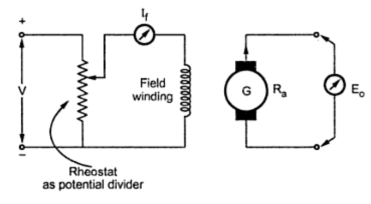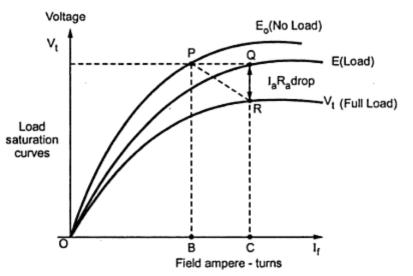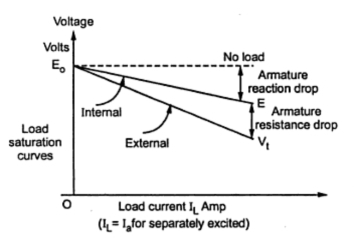### Characteristics of Separately Excited D.C. Generators

The characteristics is separately excited d.c. generator are divided into two types,
1) Magnetization   and         2) Load characteristics.
1.1 Magnetization or Open Circuit Characteristics
The arrangement to obtain this characteristics is shown in the Fig. 1.Fig. 1  Obtaining O.C.C. of separately excited generator

The rheostat as a potential driver is used to control the field current and the flux. It is varied from zero and is measured on ammeter connected.
E = (ΦPNZ)/(60A)
As If is varied, then Φ change and hence induced e.m.f. E also varies. It is measured on voltmeter connected across armature. No Load is connected to machine, hence characteristics are also called no load characteristics which is graph of E against field current If as shown in the Fig. 2. As If increases, flux Φ increases and Eo increases. After point A, saturation occurs when Φ becomes constant and hence E saturates.Fig. 2  Open circuit characteristics

This is the graph of terminal voltage Vt against field current If. When generator is loaded, armature current Ia flows and armature reaction exists. Due to this, terminal voltage Vt is less than the no load rated voltage. On no load, current Ia is zero and armature reaction is absent. Hence less number of ampere turns are required to produce rated voltage Eo .
These ampere-turns are equal to OB as shown in the Fig. 3. On load, to produce same voltage more field ampere-turns are required due to demagnetizating effect of armature reaction. These are equal to BC as shown in the Fig. 3. Similarly there is drop Ia Ra across armature resistance. Hence terminal voltage V = E - Ia Ra . This graph OR is also shown in the Fig. 3. The triangle PQR is called drop reaction triangle. Thus OP is no load saturation curve, OQ is the graph of generated voltage on load and OR is the graph of terminal voltage on load.Fig. 3  Load saturation curves

1.3 Internal and External Characteristics External
Let be the no load rated voltage which drops to E due to armature reaction on load and further drops to Vt due to armature resistance drop Ia Ra on load.
The graph of Vt against load current IL is called external characteristics while the graph of E against load current IL is called internal characteristics. These are shown in the Fig. 4. for separately excited d.c.generator. The graphs are to be plotted for constant field current. In case of separately excited d.c. generator induced e.m.f. is totally dependent on flux Φ i.e. field current If . Hence to have control over the field current, in case of separately excited d.c. generators field regulator is necessary.Fig. 4  Load characteristics